# Selina Solutions Concise Mathematics Class 6 Chapter 33 Data Handling Exercise 33(B)

Selina Solutions Concise Mathematics Class 6 Chapter 33 Data Handling Exercise 33(B) has accurate answers about the concepts, which are important for the exam. The solutions are well structured by the subject matter experts, as per the latest ICSE exam guidelines. Representing the given set of data in the form of a pictograph, is the major concept discussed here. Important formulas and shortcut methods are explained in an interactive manner to boost exam preparation among students. To ace the final exam, students can avail Selina Solutions Concise Mathematics Class 6 Chapter 33 Data Handling Exercise 33(B) PDF, from the links which are provided here.

## Selina Solutions Concise Mathematics Class 6 Chapter 33 Data Handling Exercise 33(B) Download PDF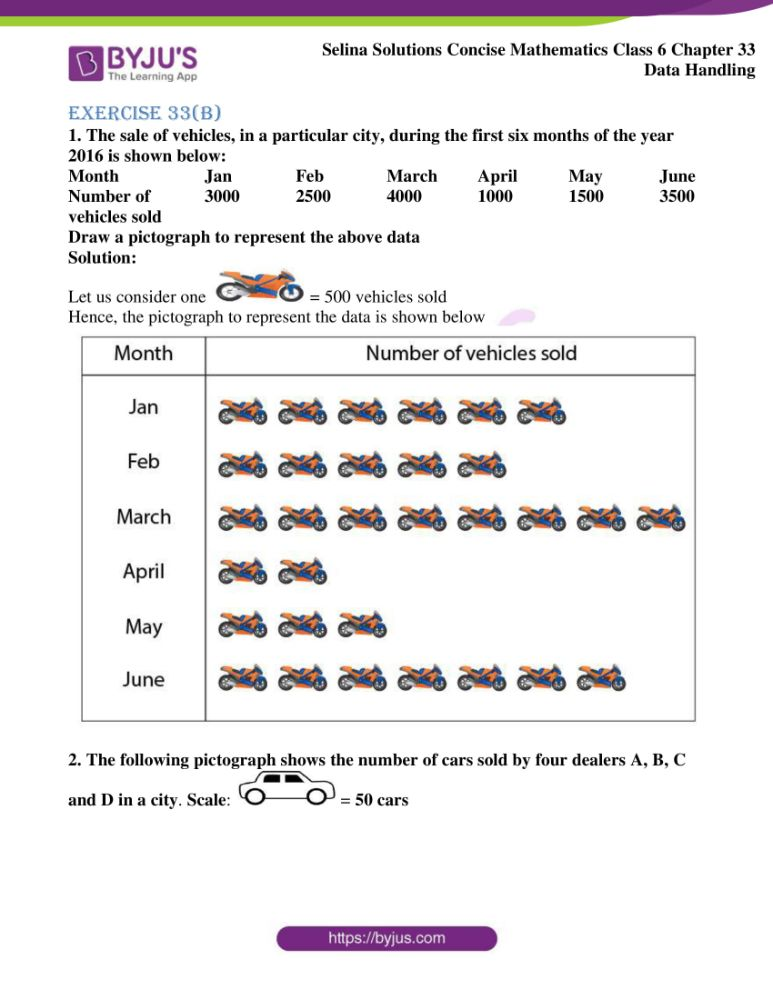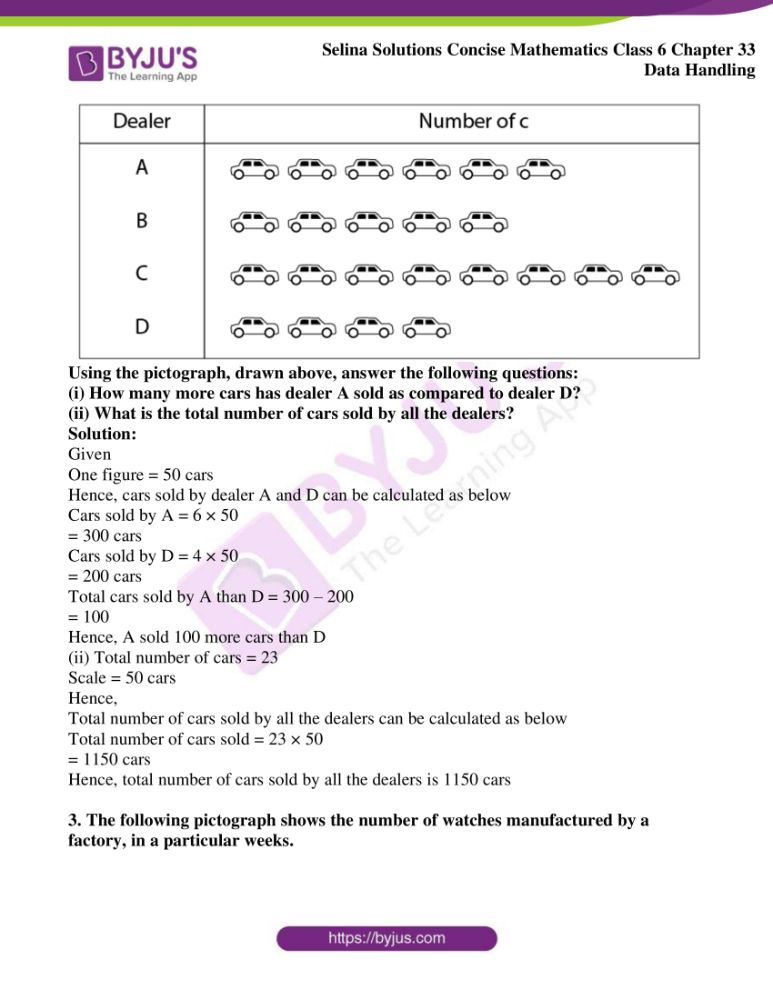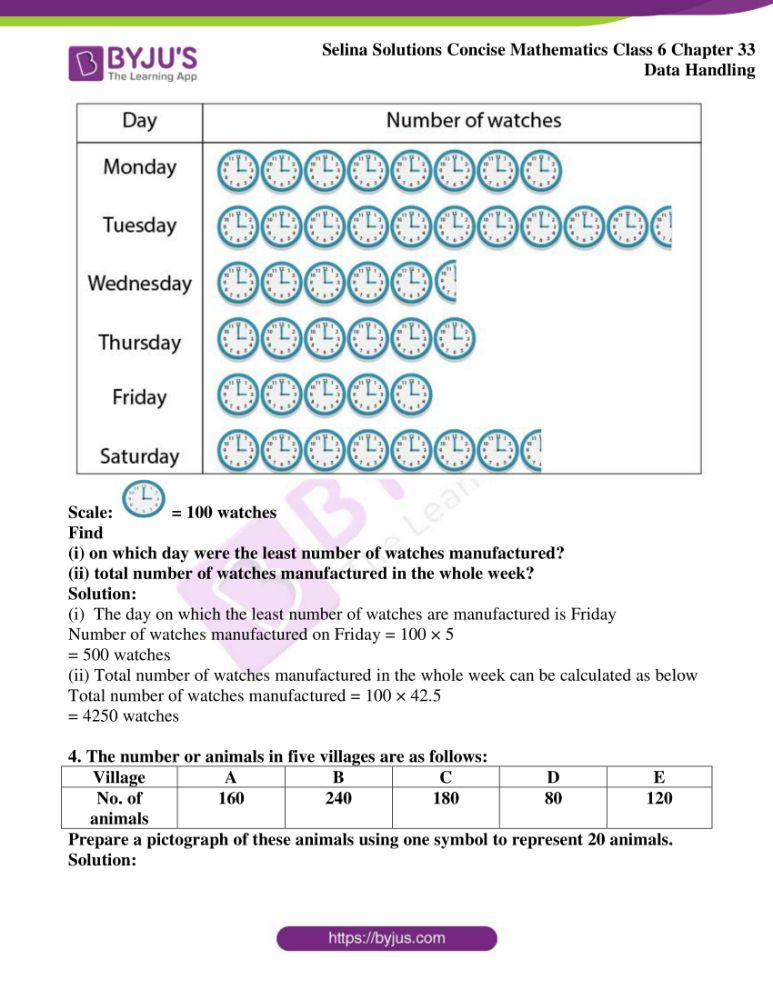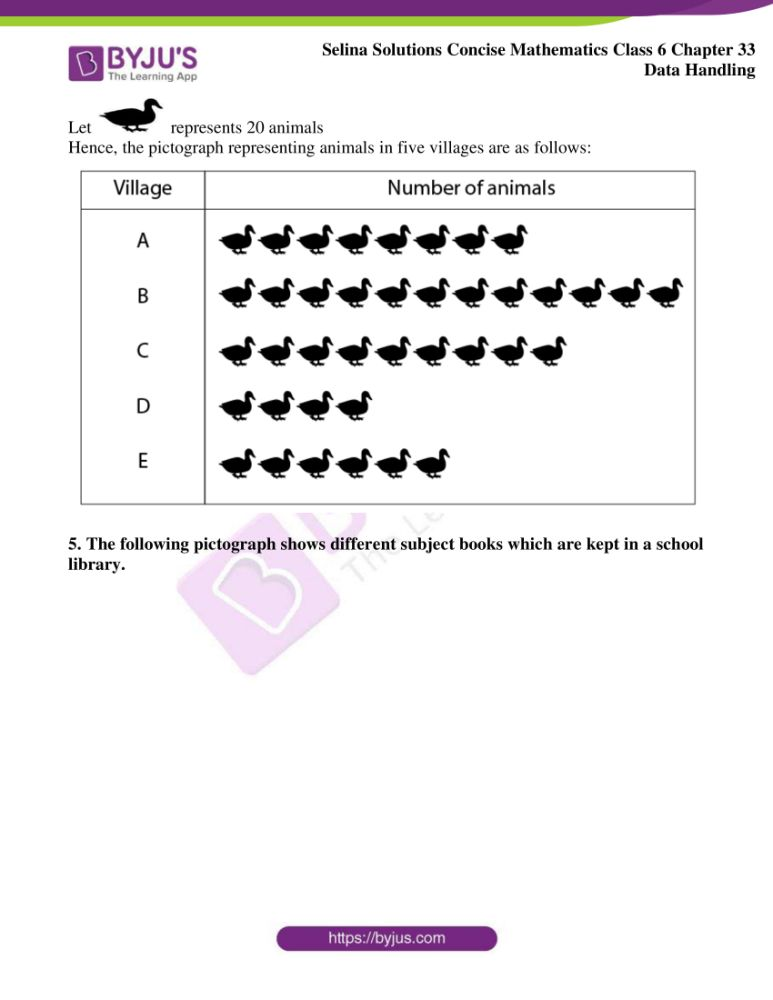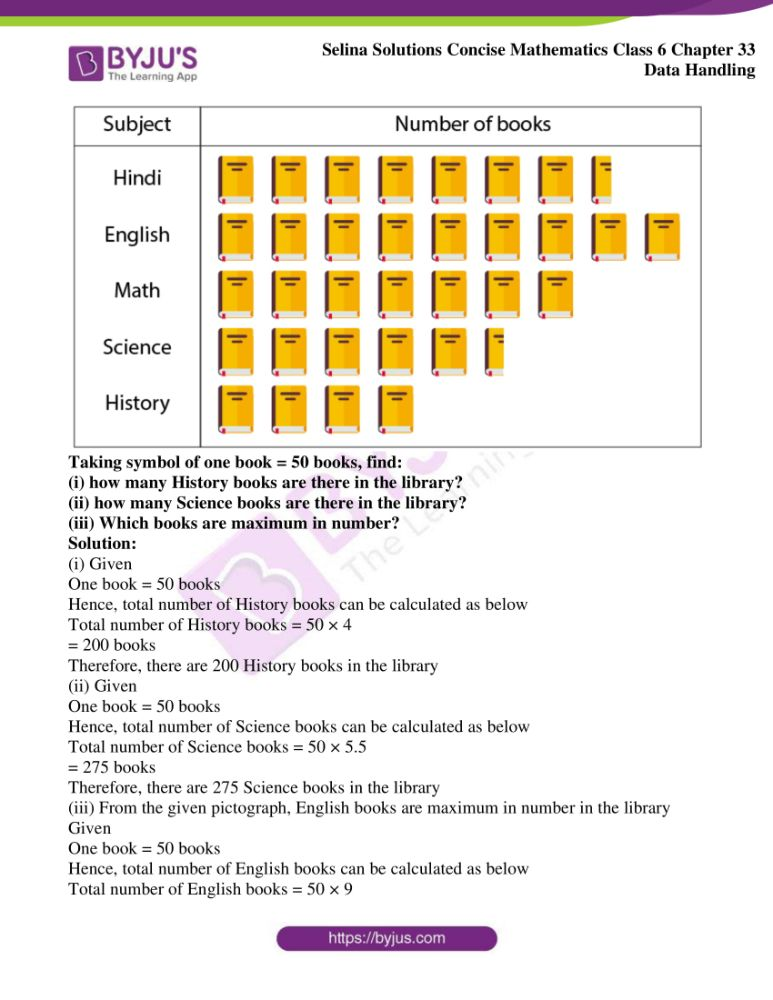### Access other exercises of Selina Solutions Concise Mathematics Class 6 Chapter 33 Data Handling

Exercise 33(A) Solutions

Exercise 33(C) Solutions

### Access Selina Solutions Concise Mathematics Class 6 Chapter 33 Data Handling Exercise 33(B)

Exercise 33(B)

1. The sale of vehicles, in a particular city, during the first six months of the year 2016 is shown below:

Month Jan Feb March April May June

Number of 3000 2500 4000 1000 1500 3500

vehicles sold

Draw a pictograph to represent the above data

Solution:

Let us consider one= 500 vehicles sold

Hence, the pictograph to represent the data is shown below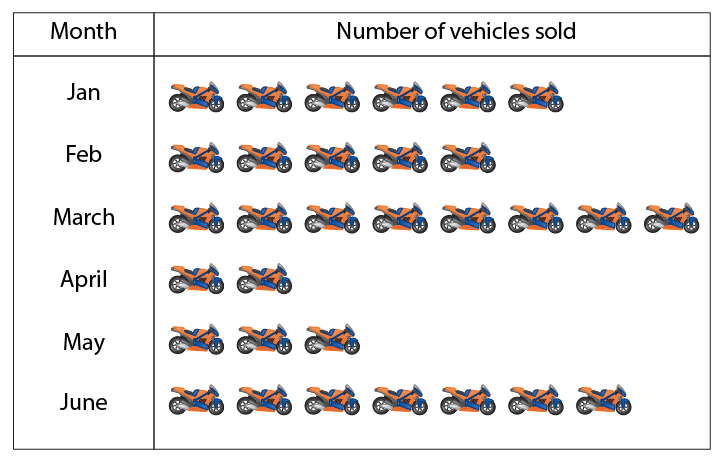2. The following pictograph shows the number of cars sold by four dealers A, B, C and D in a city. Scale: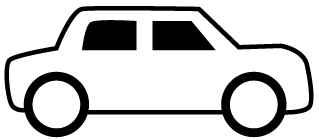= 50 cars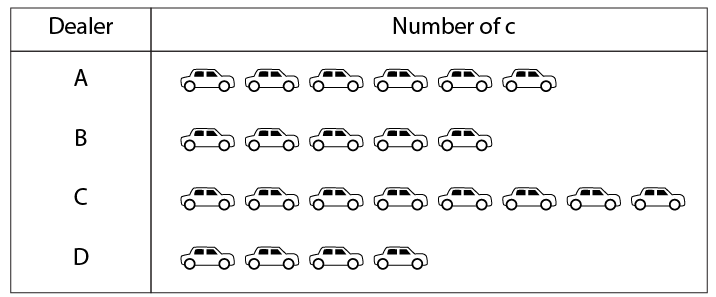Using the pictograph, drawn above, answer the following questions:

(i) How many more cars has dealer A sold as compared to dealer D?

(ii) What is the total number of cars sold by all the dealers?

Solution:

Given

One figure = 50 cars

Hence, cars sold by dealer A and D can be calculated as below

Cars sold by A = 6 × 50

= 300 cars

Cars sold by D = 4 × 50

= 200 cars

Total cars sold by A than D = 300 – 200

= 100

Hence, A sold 100 more cars than D

(ii) Total number of cars = 23

Scale = 50 cars

Hence,

Total number of cars sold by all the dealers can be calculated as below

Total number of cars sold = 23 × 50

= 1150 cars

Hence, total number of cars sold by all the dealers is 1150 cars

3. The following pictograph shows the number of watches manufactured by a factory, in a particular weeks.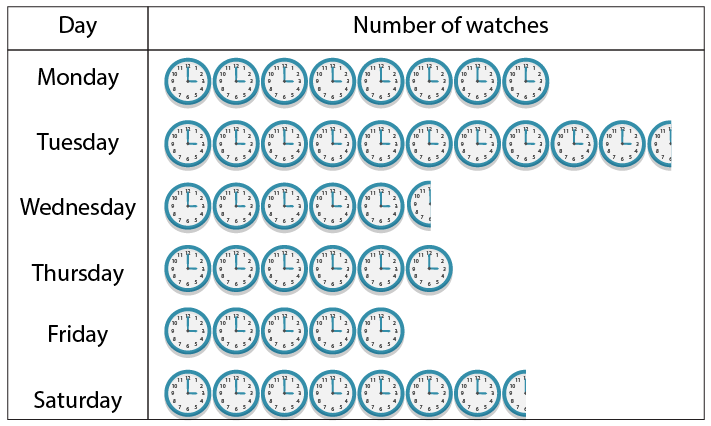Scale: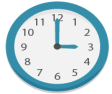= 100 watches

Find

(i) on which day were the least number of watches manufactured?

(ii) total number of watches manufactured in the whole week?

Solution:

(i) The day on which the least number of watches are manufactured is Friday

Number of watches manufactured on Friday = 100 × 5

= 500 watches

(ii) Total number of watches manufactured in the whole week can be calculated as below

Total number of watches manufactured = 100 × 42.5

= 4250 watches

4. The number or animals in five villages are as follows:

 Village A B C D E No. of animals 160 240 180 80 120

Prepare a pictograph of these animals using one symbol to represent 20 animals.

Solution:

Let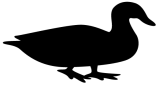represents 20 animals

Hence, the pictograph representing animals in five villages are as follows: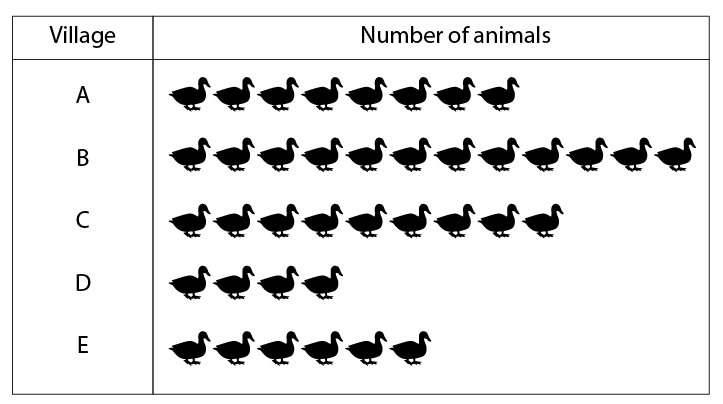5. The following pictograph shows different subject books which are kept in a school library.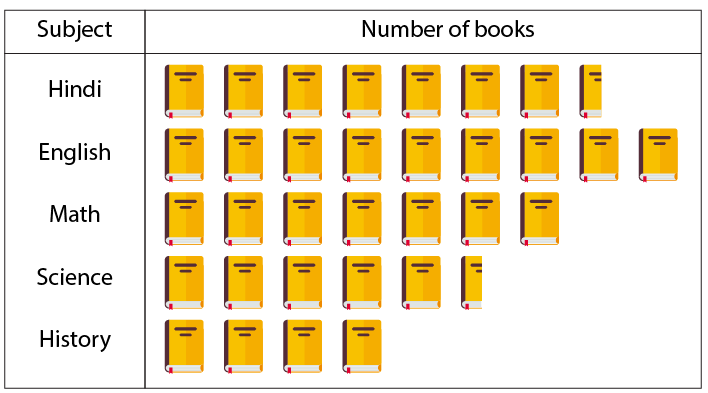Taking symbol of one book = 50 books, find:

(i) how many History books are there in the library?

(ii) how many Science books are there in the library?

(iii) Which books are maximum in number?

Solution:

(i) Given

One book = 50 books

Hence, total number of History books can be calculated as below

Total number of History books = 50 × 4

= 200 books

Therefore, there are 200 History books in the library

(ii) Given

One book = 50 books

Hence, total number of Science books can be calculated as below

Total number of Science books = 50 × 5.5

= 275 books

Therefore, there are 275 Science books in the library

(iii) From the given pictograph, English books are maximum in number in the library

Given

One book = 50 books

Hence, total number of English books can be calculated as below

Total number of English books = 50 × 9

= 450 books

Therefore, there are 450 English books in the library